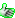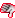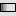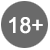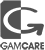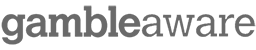# BankrollMob Forum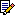BankrollMob Forum » Poker Forum » Calculating probability of a downswing

 Calculating probability of a downswing 0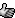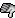I've been reading up a lot on different ways to cope with downswings and such, and I came across an excel table that had some numbers on it that shower the probability of a downswing of X buy-ins from a player with Y roi over Z number of games.

Now, this table was for regular ST SnG, but since I mainly play DoN I tried to create an equivalent table for that game but I just can't get it to work. Can anyone here figure out a way to calculate this?

Example:
Game: Double or Nothing SnG
1000 games
Winrate: 57%
ROI: 6.02%

What is the probability that such a player would hit a 10 or 20 or 50 BI downswing over the course of 1000 games? Or even 10k games?

These numbers are just imaginary, only to give you an idea of what I'm looking for.

 0I'm toying around with some different ways to approach this and I'm fairly sure I've at least figured out the formula on what the probability is of being down X number of BI's after Z number of games based in ROI (or winrate, you get the same result really). So that's something but I'm still not where I want to be.

 0I've finally managed to solve this. The equation I had to use turned out to be pretty much unsolveable by hand so had to write some code for Julia and run it through there. Something is a little bit wrong tho since with lower rake, my RoR is higher which doesn't make sense to me. We'll see if I can make it work.BankrollMob Forum » Poker Forum » Calculating probability of a downswing

Forum Rules | Support & FAQ